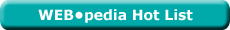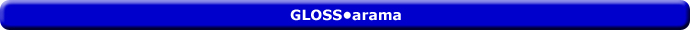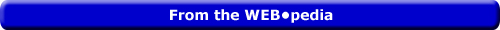Saturday  May 28, 2022
 AmosWEB means Economics with a Touch of Whimsy!LRAC CURVE: The common abbreviation for the long-run average cost curve, which is a curve depicting the per unit cost of producing a good or service in the long run when all inputs are variable. The long-run average cost curve can be derived in two ways. On is to plot long-run average cost, which is, long-run total cost divided by the quantity of output produced. at different output levels. The more common method, however, is as an envelope of an infinite number of short-run average total cost curves. Such an envelope is base on identifying the point on each short-run average total cost curve that provides the lowest possible average cost for each quantity of output. The long-run average cost curve is U-shaped, reflecting economies of scale (or increasing returns to scale) when negatively-sloped and diseconomies of scale (or decreasing returns to scale) when positively sloped. The minimum point (or range) on the LRAC curve is the minimum efficient scale.Most Viewed (Number) Visit the WEB*pediaCAPITAL ACCOUNT SURPLUS: An imbalance in a nation's balance of payments capital account in which payments received by the country for selling domestic assets exceed payments made by the country for purchasing foreign assets. In other words, investment by the domestic economy in foreign assets is greater than foreign investment in domestic assets. This is generally a desireable situation for a domestic economy. However, in the wacky world of international economics, a capital account surplus is often balanced by a current account deficit, which is not generally considered a desireable situation. If, however, the current account does not balance out the capital account, then a capital account surplus contributes to a balance of payments surplus.Recommended Citation:

CAPITAL ACCOUNT SURPLUS, AmosWEB GLOSS*arama, http://www.AmosWEB.com, AmosWEB LLC, 2000-2022. [Accessed: May 28, 2022].

Search Again?MIDPOINT ELASTICITY FORMULA

A simple technique for calculating the coefficient of elasticity by estimating the average elasticity for discrete changes in two variables. The distinguishing characteristic of this formula is that percentage changes are calculated based on the average of the initial and ending values of each variable, rather than initial values. An alternative technique is the endpoint elasticity formula.BROWN PRAGMATOX[What's This?] Today, you are likely to spend a great deal of time at a going out of business sale seeking to buy either a 50 foot extension cord or a combination CD player, clock radio, and telephone (with answering machine). Be on the lookout for malfunctioning pocket calculators.Your Complete ScopeParker Brothers, the folks who produce the Monopoly board game, prints more Monopoly money each year than real currency printed by the U.S. government."A pint of sweat saves a gallon of blood. "-- General George PattonRGDPReal Gross Domestic ProductA PEDestrian's Guide Xtra CreditTell us what you think about AmosWEB. Like what you see? Have suggestions for improvements? Let us know. Click the User Feedback link.| | | | | | | | | | |
| | | |

Thanks for visiting AmosWEB